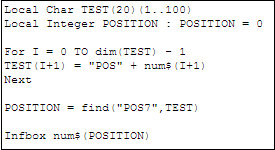# Find a particular value from a series of Numerical, Alphanumerical or Date Expressions or Variables

By | March 3, 2017

We may have come across a situation where you need to find a particular value from a series of numerical, alphanumerical or date expressions or variables. This can be achieved using “find”.

Syntax:

find(value_to_seek, list_exprg)

value_to_seek is the value that needs to be found

list_exprg is the series of numerical/alphanumerical/date expressions/variables

Example:

Consider an example where we need to find the position of string from a series of strings.Below is the response if we run the above code: